# 为WordPress添加MathJax支持

#### MathJax是什么？

MathJax是一个开源的数学公式显示脚本。其最大的优势在于可以以基于文本的方式显示页面中的数学公式。而且支持Tex和LaTex、MathML、ASCIIMathML语言来书写公式，完美支持各大主流浏览器，拥有丰富的API接口。

#### 如何安装？

MathJax的安装十分简单，只需要一句代码就可以完成配置。

<script type="text/javascript" src="http://cdn.mathjax.org/mathjax/latest/MathJax.js?config=TeX-AMS-MML_HTMLorMML"></script>

Update:

function load_mathjax(){
wp_enqueue_script('mathjax', 'https://cdnjs.cloudflare.com/ajax/libs/mathjax/2.7.5/MathJax.js?config=TeX-AMS_CHTML,Safe', array(), '1.0', false);
}

add_action('wp_enqueue_scripts', 'load_mathjax');

#### 如何使用？\begin{aligned} \nabla \times \vec{\mathbf{B}} -\, \frac1c\, \frac{\partial\vec{\mathbf{E}}}{\partial t} & = \frac{4\pi}{c}\vec{\mathbf{j}} \\ \nabla \cdot \vec{\mathbf{E}} & = 4 \pi \rho \\ \nabla \times \vec{\mathbf{E}}\, +\, \frac1c\, \frac{\partial\vec{\mathbf{B}}}{\partial t} & = \vec{\mathbf{0}} \\ \nabla \cdot \vec{\mathbf{B}} & = 0 \end{aligned}

http://www.mathjax.org/

## 《为WordPress添加MathJax支持》上有8条评论

1.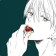梦月酱说道：

。。。。这个 不太需要Firefox 19.0Windows 7
1.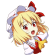hcl说道：

最近会写一点关于微积分的东西，这算是在做准备Android Webkit 4.0HTC Desire
1.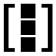大D说道：

这是要搞更高阶更牛的东西啊~~Google Chrome 25.0.1364.152Windows 7 x64 Edition
1.hcl说道：

不敢当，只是写写自己的学习心得Internet Explorer 10.0Windows 7
1.大D说道：

那也不错，哈哈Google Chrome 25.0.1364.152Windows 7 x64 Edition
2.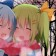酱爆灵梦说道：

数死早表示这么高级的东西伤不起Firefox 20.0Windows XP
3.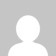鱼香肉丝说道：

为啥我加入这句话后 ，进入文章的预览模式之后 公式只看到了一眼，然后它就没了…Google Chrome 77.0.3865.90Windows 10 x64 Edition
1.hcl说道：

本文中提到的方法仅适用于文章页面的显示，预览模式下可能并不会加载之间的脚本。Firefox 69.0Mac OS X 10.14